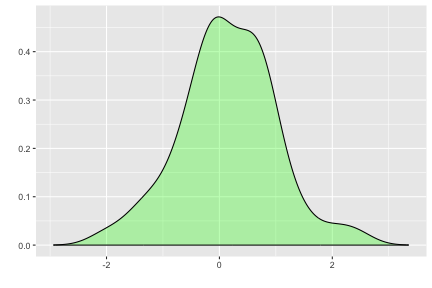This document explains how to plot probability distributions using `{ggplot2}` and `{ggfortify}`.

# Plotting Probability Distributions

`ggdistribution` is a helper function to plot Distributions in the stats package easier using `ggplot2`.

For example, plot standard normal distribution from -3 to +3:

``````library(ggfortify)
ggdistribution(dnorm, seq(-3, 3, 0.1), mean = 0, sd = 1)
``````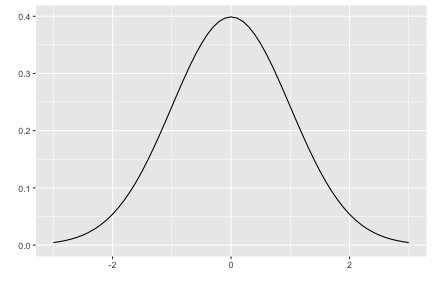`ggdistribution` accepts PDF/CDF function, sequence, and options passed to PDF/CDF function. Also, it has some options to configure how plot looks. Use `help(ggdistribution)` to check available options.

``````ggdistribution(pnorm, seq(-3, 3, 0.1), mean = 0, sd = 1, colour = 'red')
``````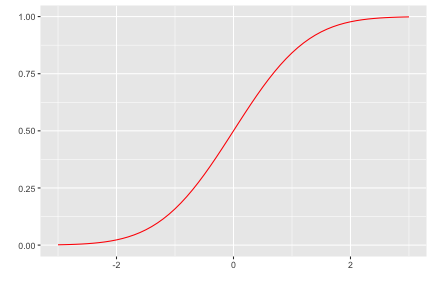``````ggdistribution(dpois, seq(0, 20), lambda = 9, fill = 'blue')
``````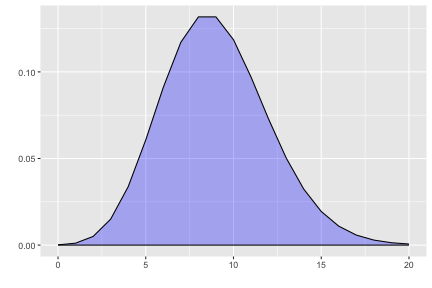If you want to plot some distributions overwrapped, use `p` keyword to pass `ggplot` instance.

``````p <- ggdistribution(dchisq, seq(0, 20, 0.1), df = 7, colour = 'blue')
p <- ggdistribution(dchisq, seq(0, 20, 0.1), df = 9, colour = 'green', p = p)
ggdistribution(dchisq, seq(0, 20, 0.1), df = 11, colour = 'red', p = p)
``````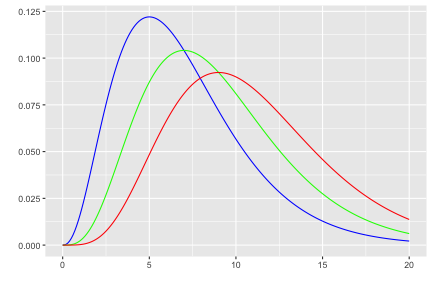# Plotting Density

Also, `autoplot` can accept `stats::density`.

``````autoplot(density(rnorm(1:50)), fill = 'green')
``````